# Introduction to Capsule Networks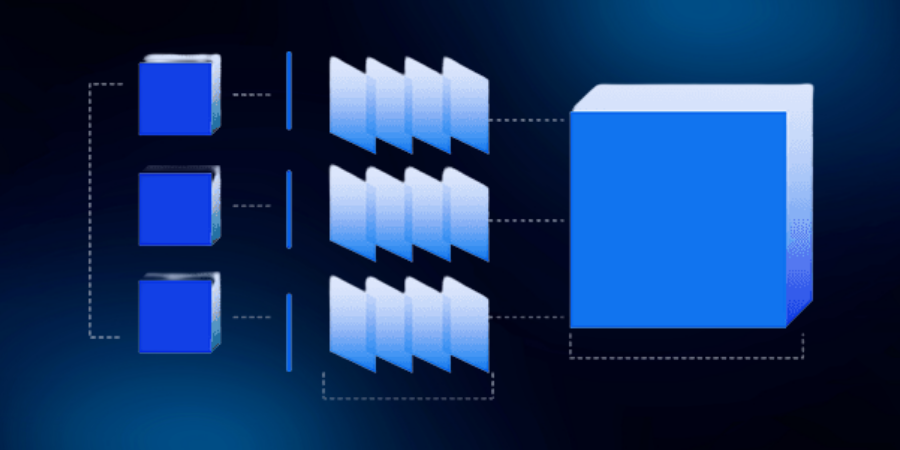S.No Lesson Title
1
2
##### Limitations of CNNs
2.1 Translational Equivariance
2.2 Human visual system and Maxpooling
2.3 Large Datasets
3
##### Capsule Networks
3.1 What are Capsules?
3.3 How Capsule Networks Work?
3.4 Routing Algorithm
4
5

### Introduction

The current workhorse which is almost used by everyone in the deep learning community are Convolutional Neural Networks. CNNs are being used in the field of image classification, object detection,semantic segmentation and many more applications. CNNs gained popularity back in the year 2012 when AlexNet won the ImageNet challenge. Since then CNNs have become the first choice in the deep learning community. But are they the best solutions for image problems?

### Limitations of CNNs

The answer to the question that I asked at the end of the previous section is NO. Let us understand the problems caused by CNNs.

#### Translational Equivariance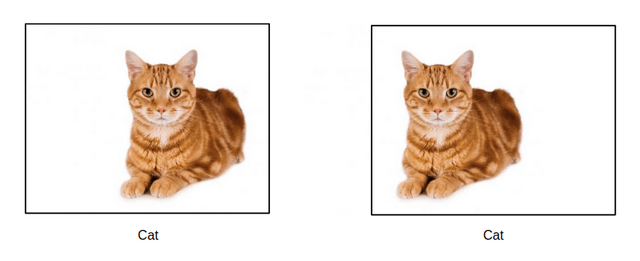The above images contain a cat but at different positions. The cat in the left image is located in the right part of the image and the cat in the right image is located in the left part of the image. If we pass these images to a CNN cat classification model, it will predict a cat in both images but it will not provide us any extra information like cat shifted to left or right etc. Here is an illustration of a CNN cat classification model.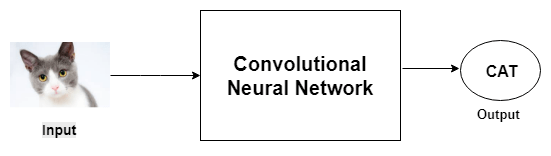Here is another illustration.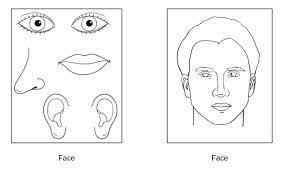The image on the left has parts of a human face - ears,eyes,nose and lips and the image on the right has a complete drawing of the human face.

If we pass these two images to a CNN face detection model, it will detect faces in both images. But the image on the left is not a face. This is the drawback of CNN's that they are translational invariant but not translational equivariant.

#### Human Visual System and Maxpooling

Most of you would have studied that CNN's represents our visual cortex system. Yes they do. But CNN's are a bad representation of the visual cortex system. When we see anything, our visual stimulus is triggered and sends the low level data to the part of the brain which can handle low level features. But CNN's do not have any such property. Infact, they generate high level semantic information from low level features through various convolution and maxpooling layers.

According to Geoffrey Hinton , "The pooling operation used in convolutional neural networks is a big mistake and the fact that it works so well is a disaster".

The fact that Hinton is pointing towards is the loss of useful information due to maxpooling.

#### Large Datasets

CNN's require a large amount of data to generalize. This requirement of a large amount of data points towards the shortcomings of backpropagation. In the case of small datasets, CNNs will fail to give a good accuracy compared to the machine learning models.

### Capsule Networks

The limitations of CNNs that I have discussed in the previous section can be solved using capsule networks. Capsule Networks were proposed by Geoffrey Hinton in his paper "Dynamic Routing Between Capsules" in 2017. Since then , capsule networks have become an active field of research. They use capsules rather than neurons.

#### What are Capsules?

Capsules are the substitutes of artificial neurons in CNN's which do the computations of their inputs and then encapsulate the information in the form of a vector. The neurons in CNN's contain information in the form of scalar quantity while the capsules contain information in the form of vectors. Their scaler term gives us the probability of an object and the direction tells us about its pose(size, position, orientation etc.). Group of these capsules form a layer and these layers result in a capsule network.

Let us understand how capsule networks can overcome the limitations of CNNs that we discussed above.

1. Translational Equivariant
2. Consider two images with the same object but different pose. If we pass these images to a capsule network, the capsule vectors will have the same probability for that object but different orientation. It can help us to understand the shift of objects inside the image and this makes capsule networks translational equivarant as well as invariant.

3. Maxpooling
4. Capsule Networks does not use any maxpooling layers and therefore saves useful information from being lost.

5. Amount of Data
6. Unlike CNN, Capsule Networks use less data for training as it saves spatial relationships with features.

#### How Capsule Networks Work?

I hope you are familiar with CNN architectures in which one layer passes information to the subsequent layers. Capsule Networks or CapsNet also follow the same flow of information. Here is the illustration.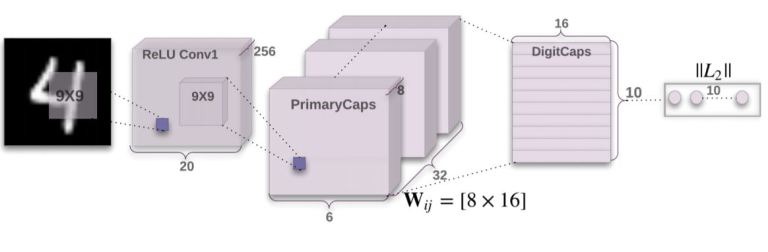The above network shows the capsule network on a MNIST dataset. Initial layers use convolution to get low level features and then pass these low level information to the primary capsule layer. The primary capsule layer reshape the convolutional layer output into capsules containing vectors of equal dimensions. The length of these vectors indicates the probability of an object and therefore we need to change the length in the range 0 to 1. This operation is known as "Squashing" in which we pass the output of capsule layers from a nonlinear function. Here is the formula for nonlinear function.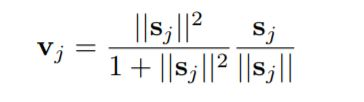Here, Sj is the input vector, ||Sj|| is the norm of the vector and Vj is the output vector. This output vector is then passed to the next layer. Capsules in the next layers are generated using a dynamic routing algorithm. Let us understand about routing algorithms.

#### Routing Algorithm

The routing algorithm in capsule networks connects capsules in consecutive layers and enables the upper level capsules to learn higher level concepts by combining the concepts of the lower level capsules. The routing algorithm defines the agreement between these capsules. The lower level capsules will send information to the higher level capsules if they agree to each other.

Let's look at a facial image as an example. If a lower layer contains four capsules, each symbolising the mouth, nose, left eye, and right eye, respectively. And if all four agree on the same face position, the output layer capsule will deliver its values indicating the presence of a face.

To generate output for the routing capsules (capsules in the higher layer), the output from the lower layer (u) is first multiplied by the weight matrix W, and then a routing coefficient C is applied. This C will identify which lower-layer capsules will transfer their output to which higher-layer capsule. Here is the mathematical expression.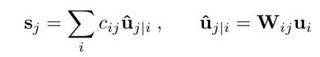The lower level capsules or child capsules send their input to higher level capsules or parent capsules to make agreement. The Capsule that makes the agreement is assigned a larger weight. Initially, the routing coefficients are all set equal depending on the number of parent capsules. Then for each parent capsule, the child capsules are multiplied with the routing coefficient and then summed up. Squashing is performed after summation to take down the values between 0 and 1. After squashing, the dot product between the child capsules and parent capsules give us the similarity. The input child capsule having highest similarity is assigned greater routing coefficient. Routing coefficients are updated as old routing coefficient plus the highest dot product. The iteration is run for user defined steps, typically 3. Here is the illustration.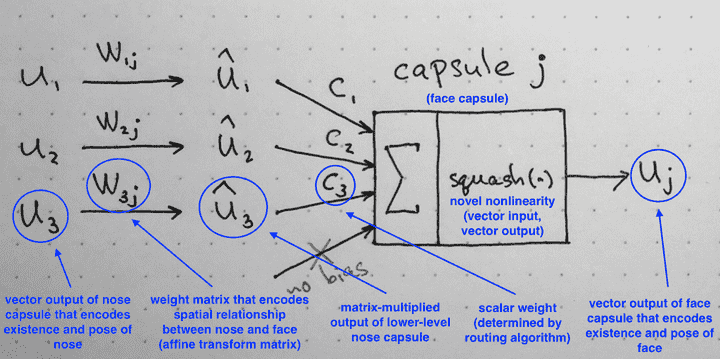### Conclusion

In this article, we have discussed the capsule networks and its architecture. If you want to deep dive into the capsule network, I would recommend you to go through the original paper given in the references.